#### Smooth Function Approximation: The TPSPLINE Procedure

The TPSPLINE procedure decomposes the regressor contributions to the mean function into parametric components and into smooth functional components. Suppose that the regressor variables are collected into the vectorand that this vector is partitioned as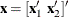. The relationship between Y and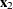is linear (parametric) and the relationship between Y and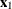is nonparametric. The TPSPLINE procedure fits models of the form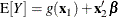The function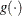can be represented as a sequence of spline basis functions.

The parameters are estimated by a penalized least squares method. The penalty is applied to the usual least squares criterion to obtain a regression estimate that fits the data well and to prevent the fit from attempting to interpolate the data (fit the data too closely).